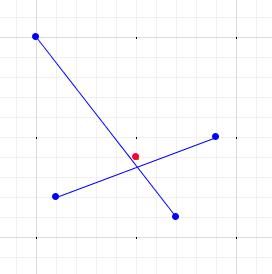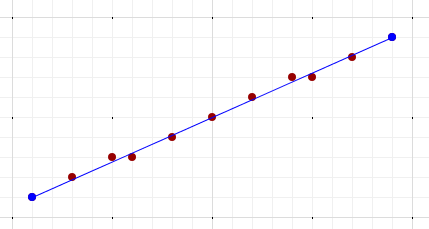# FAQ

## Design and Architecture

### Why is the C++ API not kept stable?

The C++ API is very large, and may have changes in every release. It is evolving to adopt modern C++ coding practices, and often has additions and removals as GEOS spatial algorithms get enhanced. Trying to provide a stable API would involve significant effort, and slow library evolution. The C API is significantly simpler (both in design and at the binary level), so it is much easier to keep stable.

### Why does GEOS follow JTS design?

GEOS started life as a port of JTS, so its design reflects that heritage. JTS is still evolving and improving, so keeping GEOS fairly close to the JTS design and organization makes it easier to keep porting JTS changes. However, there have been additions to GEOS which were not orginally in JTS (although in some cases those were superseded by later JTS development). Also, GEOS is intended to provide high-performance spatial algorithms, which sometimes requires using different code patterns than in Java.

## Spatial Model

### Does GEOS support computation on the geodetic ellipsoid?

No. GEOS assumes that geometries are defined in a Cartesian, planar, 2-dimensional space. Thus it cannot be used to compute accurate metrics, predicates or constructions on the geodetic ellipsoid which is usually used to model the surface of the Earth.

One way to perform geodetic computations is to project data to an appropriate planar projection using a transformation library such as PROJ. The desired geometric operations can be computed in planar space, and reprojected back to geodetic.

### Does GEOS support coordinates with measures (M)?

No, the GEOS coordinate model only supports X,Y and Z ordinates. We hope to add support for M, and also a more efficient XY coordinate storage representation.

## Robustness

### Why does `GEOSIntersects(GEOSIntersection(A, B), A) == false`?

GEOS represents geometry coordinates using IEEE-754 double-precision floating point numbers. This is a finite representation, whereas the implicit lines between vertices have infinite precision. In general it is highly unlikely that a coordinate computed via an arithmetic operation (such as a line intersection) is reported by an `intersects` test as lying exactly on the (theoretical) lines.

For example, the diagram below shows how the computed intersection point of two lines in general does not lie exactly on either line (scale exaggerrated for clarity):### Why doesn’t a computed point lie exactly on a line?

As explained in the previous question, GEOS uses finite-precision floating point arithmetic. In general coordinates computed via arithmetic operations (such as interpolation along a line) is reported by an `intersects` test as lying exactly on the (theoretical) line. This is due to both round-off error during calculations, and also because in the general case it is not possible to represent points along a line exactly using finite-precision numbers.

The diagram below shows how points interpolated along a line rarely lie exactly on the line (scale exaggerrated for clarity):